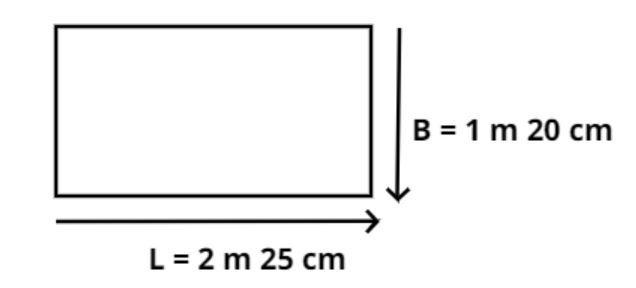Courses
Courses for Kids
Free study material
Free LIVE classes
MoreLIVE
Join Vedantu’s FREE Mastercalss

# The top of a table measures 2m 25cm by 1m 20cm. Find its area in square meters?Verified
360.6k+ views
Hint: In this question the dimension of the table which is in a rectangular shape is given and we have to find the area of this table, simple use of the basic formula for area of rectangle will help you reach the right answer.The top of the table is shown above and the measure of the table is given as 2 m 25 cm by 1m 20 cm.
Then we have to find out the area of the table.
So, let us consider that length of the table is $L = {\text{2 m 25 cm}}$
Now as we know that $1{\text{ cm = }}\dfrac{1}{{100}}{\text{ m}}$.
So length (L) = $\left( {2 + \dfrac{{25}}{{100}}} \right) = 2.25$ m.
Now let us consider that breadth of the table is $B = 1{\text{ m 20 cm}}$.
Now as we know that$1{\text{ cm = }}\dfrac{1}{{100}}{\text{ m}}$.
So breadth (B) = $\left( {1 + \dfrac{{20}}{{100}}} \right) = 1.20$ m.
So, as we see that the length and breadth of the top of the table are not equal therefore it is a rectangle table, so the area (A) of the rectangle is length multiplied by breadth.
$\therefore A = L \times B$
$\Rightarrow A = \left( {2.25{\text{ m}}} \right) \times \left( {1.20{\text{ m}}} \right) = 2.7{\text{ }}{{\text{m}}^2}$
So, this is the required area of the top of the table.

Note: Whenever we face such a type of problem the basic concept is to use the direct formula for the area of the rectangle however the key point to note is the dimensions in this questions were not given into a single unit, so always synchronize the units for all the dimensions before putting values in the dedicated formula. This will help you to get the right answer always.
Last updated date: 20th Sep 2023
Total views: 360.6k
Views today: 7.60k